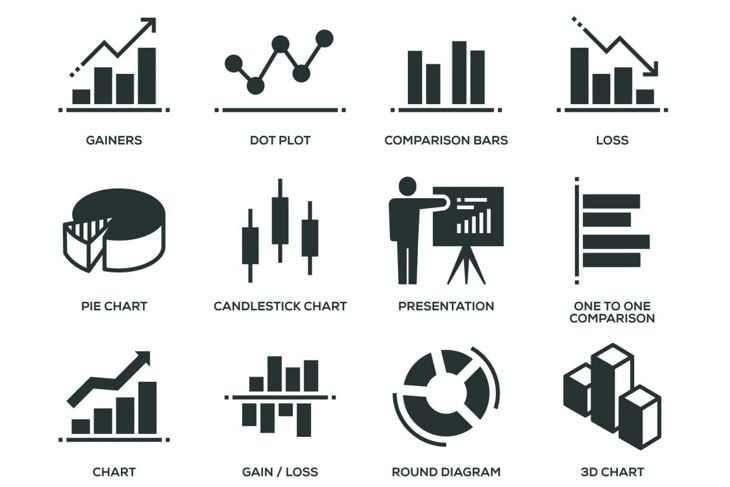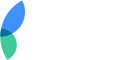Statistical analysis is important in any field, including the fields of medicine, psychology, and even business. Without a deep statistical analysis of your facts and figures, it is impossible to know what decisions to make regarding your business or research projects. The P-Value is the range of statistical significance and validity that a particular statistic shows, given the various factors surrounding it. In other words, it shows how important the stat is in terms of decision-making and value.

## What is a P-value exactly?

Before we attempt to illustrate how to calculate the P-value of a null hypothesis, let's first look at the definition of a P-value. A P-value is the amount of marginal significance of a statistical fact. So that means that, when we speak of P-values, we are really talking about statistics that are barely within the significant range.

When dealing with null hypotheses, it increases the importance of it because it means that the null hypothesis is not correct.SolStock / Getty Images

## What is a null hypothesis?

To understand P-values, we must also understand what is meant by a null hypothesis. A null hypothesis is the degree to which there is no difference between a certain population in a statistical study. It can also point directly to the fact that the statistic is not significant. This would void the statistic as unimportant to the study, and it would be thrown out.

Therefore, it is critical to understand how to calculate the P-value so that there is no question of the validity and significance of a given statistic.YakobchukOlena / Getty Images

## Purpose of the P-Value in Hypothesis Testing

To simplify things, we should note that the real purpose of the P-value in hypothesis testing is to attempt to throw out the idea that there is no significance in the facts that are being studied to a given population. Statistics always relies on segmenting groups of people or entities that are studied in a certain setting to determine the statistical trends.

The P-value is put in with the other statistical research so that people will know the information is accurate and applicable to the given population and results.

Without it, there would always be ambivalence on whether the study is valid in statistical terms and whether it holds water in what it attempts to prove.SARINYAPINNGAM / Getty Images

## Question vs. Hypothesis

Another issue that should be understood is the difference between a question and a hypothesis. For example, the question in a research study might be:

"Does a training program in driver safety result in a decline in accident rates?"

This is the question we are researching. But the hypothesis might be:

"People who take an accident prevention course will have a lower rate of accidents than those who do not."

As you can see, the hypothesis is written as a statement that you seek to prove, based on the question you are attempting to answer with facts and statistics.

Therefore, if your P-value is too high, it indicates that the null hypothesis (that people who take accident courses do not have fewer accidents) is true.PeopleImages / Getty Images

## The Calculation Process

To calculate the P-value, follow these steps:

• Determine your study's expected results.
• Determine your study's observed results (what really happened).
• Find your experiment's degrees of freedom. (number of categories)
• Compare the expected results to observed results using the chi-square formula.
• Choose a significance level.
• Use a chi-square table to determine your p-value.
• Decide whether to accept or reject your null hypothesis.NicoElNino / Getty Images

## What is the standard of significance?

The higher the P-value, the lower the incidence of accuracy that the study was statistically significant. Generally speaking, P-values that fall under the +-.05 value are still considered statistically significant. The lower the P-value, the more significant the study is.

The P-value is so important that it is often (and almost always) included in the final report about the research so that researchers and others reading the results will understand the results were statistically significant.

This also stops a lot of debate among those on the other side who claim the research is biased or skewed or not based on scientific methods.z_wei / Getty Images

## How to Figure the Results of Your Data

Take your raw data and determine the mean of your information that you have gathered. Perform both a left tail and a right tail test in a standard distribution model. These are tests that are performed to show the distributions of the data to determine an average or a mean.

You are looking at trends that are logical within your research that indicate the statistical significance. If your left or right tail test prove to be true, this may indicate a definite trend in your sampled data.

As long as your results in testing disprove the null hypothesis, your original hypothesis is probably significant.PeopleImages / Getty Images

## P-value is the probability that you are correct.

In the simplest terms, your P-value shows that your hypothesis is correct, as proven by the rejection of the null hypothesis. You are looking for numbers that indicate your research hypothesis is correct.

The key as a researcher is to look at any trends that are 1 or 2 standard deviations to the left or right, along with any other results that seem to be extreme when plotted on a distribution graph.Enis Aksoy / Getty Images

## Do the math.

The process of determining the P-value of a statistical study is listed below. Keep in mind, however, that several steps may need to be included other than these if your hypothesis involves various facts or populations.

1. Do a left tail test in a normal distribution.

2. Take the z value and plug in the formula.

3. Find the probability that z is less than 1.34, for example where z=1.34.

4. Perform the right tail test in the same way and plug in the variables.

5. Use your distribution table to determine the p-value.ra2studio / Getty Images

## Applying What You've Learned About P-Values

Statistics is about math, but that doesn't mean you should not apply some practical common sense.

In the end, statistics is about research, and that's why the p-value is so valuable. Once you figure the p-value (the probability that the null hypothesis is wrong), you can present your research with confidence, noting the p-values as proof that you are more likely to be right than wrong.

Kind of a neat way to let numbers back you up, huh?marchmeena29 / Getty Images

## Popular Now on Facty

### Disclaimer

This site offers information designed for educational purposes only. The information on this Website is not intended to be comprehensive, nor does it constitute advice or our recommendation in any way. We attempt to ensure that the content is current and accurate but we do not guarantee its currency and accuracy. You should carry out your own research and/or seek your own advice before acting or relying on any of the information on this Website.Do you want to advertise on Facty.com?
Let’s talk about this! Contact us!